There are quite a lot of articles about how to import data from CSV file to Laravel database. But none of them touches the case of matching the fields from header to actual database columns, and sometimes we’re not sure what columns are passed in user’s CSV. So this tutorial will provide a broader picture on CSV import, with example project available on Github. Let’s go!

## What we’re building here

Let’s start with the end result in mind – I will show you two screenshots.

This one represents the CSV upload form: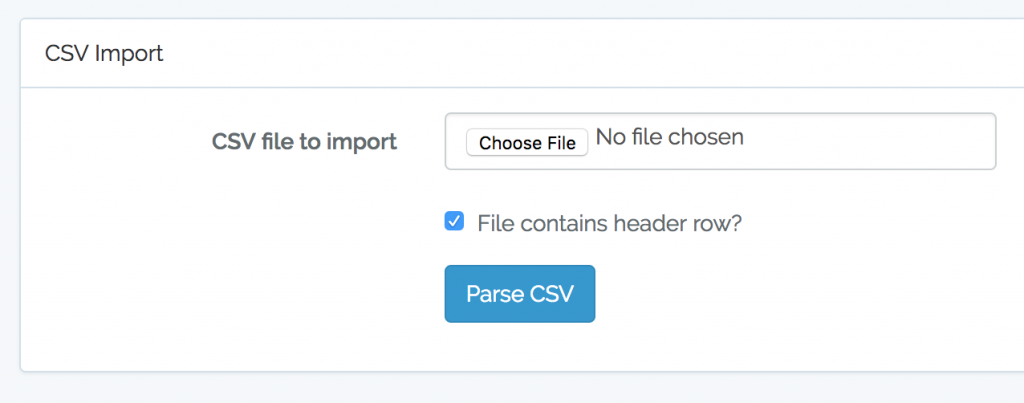Next – we parse that CSV and give the user a choice of which columns represent which database field: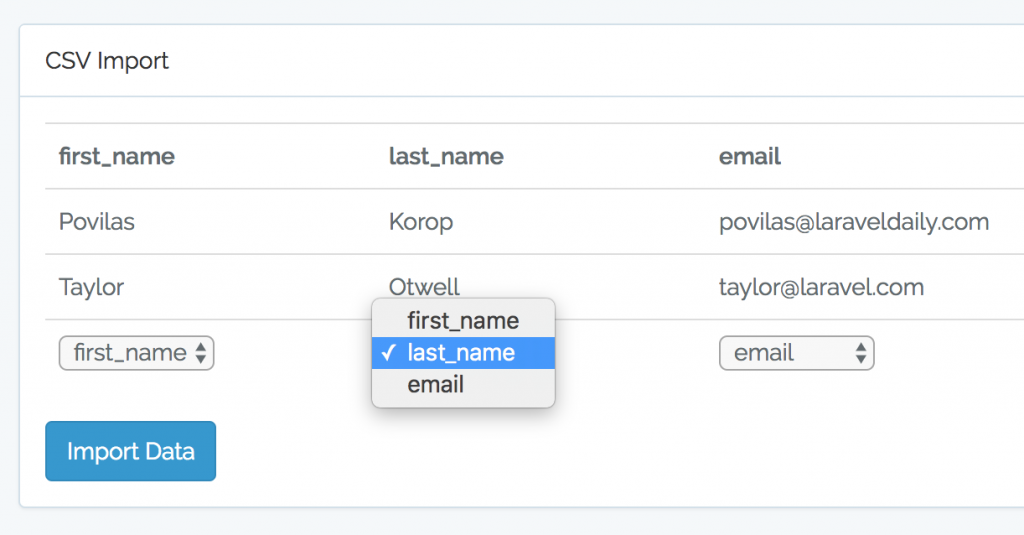And only then successful import message screen.

Now, let’s build it step-by-step.

If you prefer a video version of this tutorial, I’ve prepared it here:

## CSV file and database structure

Let’s take a simple example of CSV file with some contact details of people – just first name, last name and email. Here’s our CSV: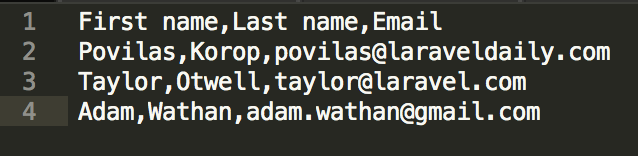Next – we create a migration for the table:

```public function up()
{
Schema::create('contacts', function (Blueprint \$table) {
\$table->increments('id');
\$table->string('first_name');
\$table->string('last_name');
\$table->string('email');
\$table->timestamps();
});
}
```

And the model app/Contact.php with fillables:

```class Contact extends Model
{
public \$fillable = ['first_name', 'last_name', 'email'];
}
```

## Import form

This part is pretty easy. I’ve taken a new Laravel 5.5 project, ran php artisan make:auth and copied login.blade.php view into a new resources/views/import.blade.php which looks like this:

```@extends('layouts.app')

@section('content')
<div class="container">
<div class="row">
<div class="col-md-8 col-md-offset-2">
<div class="panel panel-default">

<div class="panel-body">
<form class="form-horizontal" method="POST" action="{{ route('import_parse') }}" enctype="multipart/form-data">
{{ csrf_field() }}

<div class="form-group{{ \$errors->has('csv_file') ? ' has-error' : '' }}">
<label for="csv_file" class="col-md-4 control-label">CSV file to import</label>

<div class="col-md-6">
<input id="csv_file" type="file" class="form-control" name="csv_file" required>

@if (\$errors->has('csv_file'))
<span class="help-block">
<strong>{{ \$errors->first('csv_file') }}</strong>
</span>
@endif
</div>
</div>

<div class="form-group">
<div class="col-md-6 col-md-offset-4">
<div class="checkbox">
<label>
</label>
</div>
</div>
</div>

<div class="form-group">
<div class="col-md-8 col-md-offset-4">
<button type="submit" class="btn btn-primary">
Parse CSV
</button>
</div>
</div>
</form>
</div>
</div>
</div>
</div>
</div>
@endsection
```

Nothing too fancy here – csv_file field for file upload and header checkbox, user will tell us if their CSV contains a header row.Hey, this is Povilas! Do you like my article about Laravel?
We can also help to generate Laravel code!

## Routes and Controller

Our routes/web.php looks like this:

```Route::get('/', 'ImportController@getImport')->name('import');
Route::post('/import_parse', 'ImportController@parseImport')->name('import_parse');
Route::post('/import_process', 'ImportController@processImport')->name('import_process');
```

So three actions: import form, parsing fields screen, and processing with success (or not).

The first one looks really simple:

```class ImportController extends Controller
{

public function getImport()
{
return view('import');
}
```

That’s it, no data to pass to the view, no more logic here. For now.

Step further – let’s actually parse our CSV.
I will show the method to you step-by-step.

```public function parseImport(CsvImportRequest \$request)
{
\$path = \$request->file('csv_file')->getRealPath();
// To be continued...
}
```

The first part – actually getting the CSV file, it’s a one-liner in Laravel. With that, comes the Request file for validation, which is also pretty simple, we just need to make sure CSV is uploaded:

```class CsvImportRequest extends FormRequest
{
public function authorize()
{
return true;
}

public function rules()
{
return [
'csv_file' => 'required|file'
];
}
}
```

Next, how to parse the data. We will have two scenarios here – with or without header row.

## Parsing CSV without header row

Let’s take care of without header first. Imagine we have this CSV: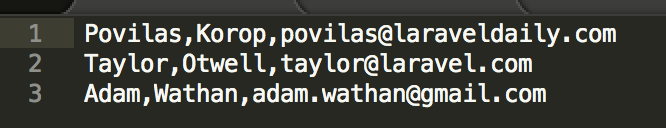Then parsing CSV into array is also a one-liner.

```public function parseImport(CsvImportRequest \$request)
{
\$path = \$request->file('csv_file')->getRealPath();
\$data = array_map('str_getcsv', file(\$path));
// To be continued...
}
```

So we will have \$data array of rows, where each row will have array of columns. Now, we can represent is as a table and give user a choice of fields.

```public function parseImport(CsvImportRequest \$request)
{
\$path = \$request->file('csv_file')->getRealPath();
\$data = array_map('str_getcsv', file(\$path));
\$csv_data = array_slice(\$data, 0, 2);
return view('import_fields', compact('csv_data'));
}
```

I’m doing the slicing of only first two lines cause they are enough to show, so user would understand which value is in which column. No need to show whole CSV here.

Now, this is how our import_fields.blade.php looks like:

```<form class="form-horizontal" method="POST" action="{{ route('import_process') }}">
{{ csrf_field() }}

<table class="table">
@foreach (\$csv_data as \$row)
<tr>
@foreach (\$row as \$key => \$value)
<td>{{ \$value }}</td>
@endforeach
</tr>
@endforeach
<tr>
@foreach (\$csv_data as \$key => \$value)
<td>
<select name="fields[{{ \$key }}]">
@foreach (config('app.db_fields') as \$db_field)
<option value="{{ \$loop->index }}">{{ \$db_field }}</option>
@endforeach
</select>
</td>
@endforeach
</tr>
</table>

<button type="submit" class="btn btn-primary">
Import Data
</button>
</form>
```

And here we have something like this – same screenshot from above:A few comments here. As you can see, we have another form which shows the table of first two rows of CSV and then one row with dropdown for each column.

For simplicity, we save the options for the columns as array in config/app.php:

```'db_fields' => [
'first_name',
'last_name',
'email'
]
```

So we call then like config(‘app.db_fields’) and loop through them.

Also, we’re using \$loop->index structure to set the value of the field. Read more about The Loop Variable here.

## Saving data temporarily

Now, in order to then process the data, we need to store it somewhere – remember, in the table we show only two first rows.

There are various ways to do that, I’ve chosen to use a separate database table for CSV files:

```Schema::create('csv_data', function (Blueprint \$table) {
\$table->increments('id');
\$table->string('csv_filename');
\$table->longText('csv_data');
\$table->timestamps();
});
```

And a simple model app/CsvData.php:

```class CsvData extends Model
{
protected \$table = 'csv_data';
protected \$fillable = ['csv_filename', 'csv_header', 'csv_data'];
}
```

So if we go back to the controller, we need to store full data in this table – here’s how it will look now:

```public function parseImport(CsvImportRequest \$request)
{
\$path = \$request->file('csv_file')->getRealPath();
\$data = array_map('str_getcsv', file(\$path));

\$csv_data_file = CsvData::create([
'csv_filename' => \$request->file('csv_file')->getClientOriginalName(),
'csv_data' => json_encode(\$data)
]);

\$csv_data = array_slice(\$data, 0, 2);
return view('import_fields', compact('csv_data', 'csv_data_file'));
}
```

We’re saving the file’s data in the database with json_encode(), and passing the result to the view. Then, in our form import_fields.blade.php, we’re showing which file we want to process, by specifying its ID as a hidden field.

```<form class="form-horizontal" method="POST" action="{{ route('import_process') }}">
{{ csrf_field() }}
<input type="hidden" name="csv_data_file_id" value="{{ \$csv_data_file->id }}" />
```

Now it’s time to actually process the data.

## Storing data into DB

Actually, this part is also pretty easy – we just need to match the dropdown values with actual column values. Here’s how it looks:

```public function processImport(Request \$request)
{
\$data = CsvData::find(\$request->csv_data_file_id);
\$csv_data = json_decode(\$data->csv_data, true);
foreach (\$csv_data as \$row) {
\$contact = new Contact();
foreach (config('app.db_fields') as \$index => \$field) {
\$contact->\$field = \$row[\$request->fields[\$index]];
}
\$contact->save();
}

return view('import_success');
}
```

We’re getting the data from DB, do json_decode() (second parameter true gives us an array result), and then loop through those.

The most difficult part is probably this line:

```\$contact->\$field = \$row[\$request->fields[\$index]];
```

Here we’re assigning a value to the property, according to the dropdown value from the fields table.

And that’s it – view success!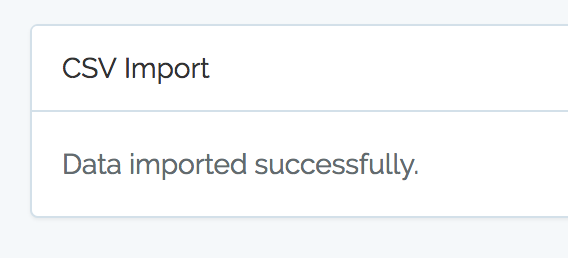## Importing data with header row

Now, if we have a header row, we can do more with parsing – we can actually guess which field the column represents!

Not only that, we can actually take the columns and assign the array keys to them, so instead of this:

```\$row = 'Taylor';
\$row = 'Otwell';
\$row = 'taylor@laravel.com';
```

We would have this structure:

```\$row['first_name'] = 'Taylor';
\$row['last_name'] = 'Otwell';
\$row['email'] = 'taylor@laravel.com';
```

We could do it parsing CSV’s first row manually, but there’s a package that can help us to do it quicker: maatwebsite/excel. It is actually used for not only importing, but exporting and manipulating Excel data, but we will take only small part of it – loading and parsing CSV.

So we’re doing:

```composer require "maatwebsite/excel:~2.1.0"
```

And then we can use it in our ImportController.
Notice: from Laravel 5.5 there’s no need to add provider/alias into config/app.php. Awesome!

```use Maatwebsite\Excel\Facades\Excel;
```

And then here’s our “upgraded” parse method:

```public function parseImport(CsvImportRequest \$request)
{

\$path = \$request->file('csv_file')->getRealPath();

} else {
\$data = array_map('str_getcsv', file(\$path));
}

if (count(\$data) > 0) {
foreach (\$data as \$key => \$value) {
}
}
\$csv_data = array_slice(\$data, 0, 2);

\$csv_data_file = CsvData::create([
'csv_filename' => \$request->file('csv_file')->getClientOriginalName(),
'csv_data' => json_encode(\$data)
]);
} else {
return redirect()->back();
}

return view('import_fields', compact( 'csv_header_fields', 'csv_data', 'csv_data_file'));

}
```

I’ve highlighted the new parts that were added:

• Parsing the data with Excel::load

The most awesome part of this Excel package, is that it will automatically change header’s values into sluggables. So instead of \$row[‘First name’] = ‘Taylor’; we will have \$data[‘first_name’] = ‘Taylor’;. That’s probably the main advantage of using it – this way we can try to guess the columns.

And that’s exactly what we will do in import_fields.blade.php:

```<form class="form-horizontal" method="POST" action="{{ route('import_process') }}">
{{ csrf_field() }}
<input type="hidden" name="csv_data_file_id" value="{{ \$csv_data_file->id }}" />

<table class="table">
<tr>
@endforeach
</tr>
@endif
@foreach (\$csv_data as \$row)
<tr>
@foreach (\$row as \$key => \$value)
<td>{{ \$value }}</td>
@endforeach
</tr>
@endforeach
<tr>
@foreach (\$csv_data as \$key => \$value)
<td>
<select name="fields[{{ \$key }}]">
@foreach (config('app.db_fields') as \$db_field)
<option value="{{ (\Request::has('header')) ? \$db_field : \$loop->index }}"
@if (\$key === \$db_field) selected @endif>{{ \$db_field }}</option>
@endforeach
</select>
</td>
@endforeach
</tr>
</table>

<button type="submit" class="btn btn-primary">
Import Data
</button>
</form>
```

Again, I’ve highlighted the new code here – basically, we’re using column names as dropdown values now, and also trying to guess if CSV column name is equal to our database column name – then automatically suggest the dropdown value.

Finally, processing that import doesn’t look that different – another if-statement to check whether we have a header row.

```public function processImport(Request \$request)
{
\$data = CsvData::find(\$request->csv_data_file_id);
\$csv_data = json_decode(\$data->csv_data, true);
foreach (\$csv_data as \$row) {
\$contact = new Contact();
foreach (config('app.db_fields') as \$index => \$field) {
\$contact->\$field = \$row[\$request->fields[\$field]];
} else {
\$contact->\$field = \$row[\$request->fields[\$index]];
}
}
\$contact->save();
}

return view('import_success');
}
```

So, that’s it, project is done, please use it and expand however you want.
Here’s the link to GitHub repository: https://github.com/LaravelDaily/Laravel-Import-CSV-Demo

As a final notice, you may want to play around with more validation rules, bigger CSV files and see how quicker it is to parse them with native PHP instead of Excel package, or maybe you will find even more efficient way? Let me know in the comments.